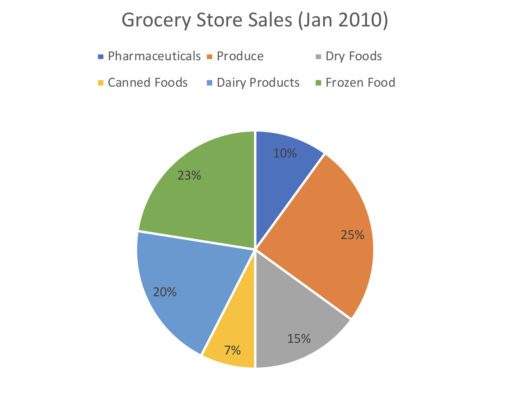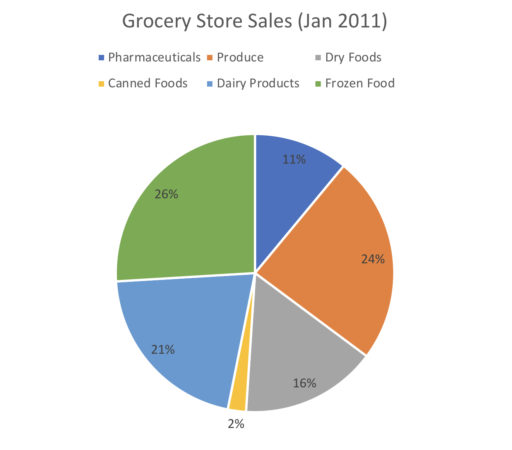[latexpage]

Circle graphs (also called pie charts) let you see the relative amounts of different categories. For example, if you run a local grocery store and want to see where your sales are coming from (perhaps because you are considering whether to re-allocate floor space), you might look at a chart like the following:and conclude that as most of your sales come from produce, you may want to allocate more space to new kinds of produce.

Now, when reading a circle graph, the percentages of the different sections will generally be labelled as in the above. So circle graph tells you that in January of 2010, 23% of all sales were from frozen foods, 10% of all sales were from pharmaceuticals, and so on.

Now, if you are given the actual value (as opposed to the proportion) of any category, you can find the value for each category. So, for example:

Example 1

Suppose that in January of 2010, the grocery store sold \$23,000 worth of frozen foods. How many dollars worth of canned foods did they sell? We can also use circle graphs to determine various trends. For example, by comparing the following charts:we can conclude that the proportion of revenue from canned foods drastically shrunk from January 2010 to 2011. Example 2 From January 2010 to January 2011, which category grew the most as a proportion of total sales? Now, if we know the actual value of some category in 2010 and the actual value of some category in 2011, we can calculate the value of each category and 2010 and 2011. Thus, we can calculate the absolute increase in revenue for any particular category as follows: Example 3 Suppose in January 2010, the total amount of goods sold was \$200,000. In January 2011, the amount of canned foods sold was \$6,000. Find the absolute change from 2010 to 2011 in the amount of dairy products sold. Practice Problems 1. Suppose produce sales were, in absolute terms, \$10,000 greater than pharmaceutical sales in January 2010. What was the total amount of sales for all goods?2. Overall sales in January 2010 were \\$100,000. How much more revenue was generated by dry foods as compared to canned foods?3. Suppose dry foods in January 2010 were twice as large, in absolute terms, as pharmaceutical sales in January 2011. What is the ratio of overall sales from January 2010 to January 2011?